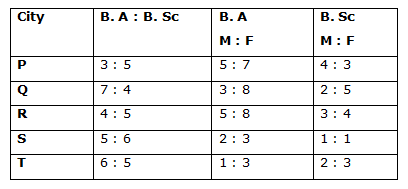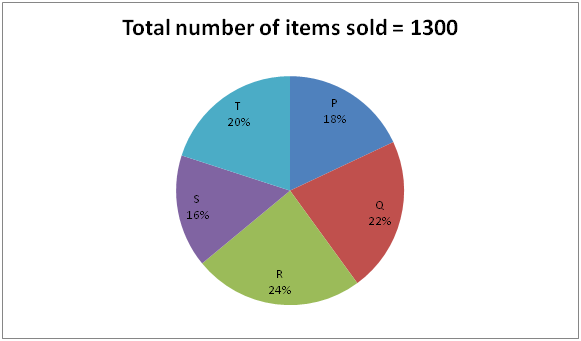# Quantitative Aptitude Questions (Data Interpretation) for NIACL Assistant Mains 2018 Day-210

Dear Readers, NIACL Assistant is conducting Online Examination for the recruitment of Assistant. To enrich your preparation here we have providing new series of Data Interpretation – Quantitative Aptitude Questions. Candidates those who are appearing in NIACL Assistant Mains Exams can practice these Quantitative Aptitude average questions daily and make your preparation effective.

Directions (Q. 1 – 5) Study the following information carefully and answer the given questions:

The following table shows the proportion of students passed from different streams in graduation from different city and also the ratio of males and females among them.1) If the total number of males who passed in B. Sc from City R is 1275, then find the total number of students passed in B. A from City R?

a) 2380

b) 3256

c) 2178

d) 3384

e) None of these

2) If the total number of females who passed in B. A from City P is 1113, then find the difference between the total number of students passed in B. A and that of B. Sc in City P?

a) 1094

b) 1180

c) 1986

d) 1272

e) None of these

3) If the total number of students passed from City S is 2794, then the total number of females passed in B. A from City S is what percentage of total number of males passed in B. Sc from City S?

a) 300 %

b) 120 %

c) 100 %

d) 140 %

e) None of these

4) The number of students passed in B. A from City T is 1716. Find the ratio between the total number of females who passed in B. A from City T to that of total number of males who passed in B. Sc from City T?

a) 1287: 572

b) 1331: 557

c) 1089: 452

d) 956: 369

e) None of these

5) The number of females who passed in B. A from City Q is approximately more/less than the number of males who passed in B. A from City Q?

a) 145 % less

b) 167 % more

c) 152 % less

d) 173 % more

e) 134 % less

Directions (Q. 6 – 10) Study the following information carefully and answer the given questions:

The following pie chart shows the total no of items sold by five different shops P, Q, R, S and T in a particular month. They sell two different items x and y.6) What is the central angle corresponding to the total number of items sold by shop Q and S together?

a) 127.60

b) 114.40

c) 136.80

d) 142.20

e) None of these

7) If the total number of item X sold by Shop R to that by Shop T is in the ratio of 3 : 4 and the total number of item Y sold by Shop T is 108, then find the total number of item X sold by shop R?

a) 126

b) 114

c) 132

d) 98

e) None of these

8) If the total number of item Y sold by shop Q is 112 and the ratio of item X to Y sold by shop P is 5 : 4, then find the difference between the total number of item X sold by Shop Q to that of total number of item Y sold by Shop P?

a) 86

b) 94

c) 70

e) 62

e) None of these

9) If the total number of items sold by Shop S is 40 % of total number of items purchased by Shop S, then find the total number of unsold items in Shop S?

a) 312

b) 258

c) 376

d) 404

e) None of these

10) Total number of items sold by shop Q and T together is approximately what percentage more/less than the total number of items sold by shop R and S together?

a) 8 % less

b) 12 % more

c) 5 % more

d) 17 % less

e) 5 % less

Direction (1-5) :

Explanation:

The total number of males who passed in B. Sc from City R= 1275

3’s = 1275

1’s = 425

Total number of students passed in B. Sc from City R = 7’s = 2975

Total number of students passed in B. A from City R = 2975*(4/5) = 2380

Explanation:

The total number of females who passed in B. A from City P= 1113

7’s = 1113

1’s = 159

Total number of students passed in B. A from City P = 159*12 = 1908

Total number of students passed in B. Sc from City P = 1908*(5/3) = 3180

Required difference = 1272

Explanation:

The total number of students passed from City S = 2794

11’s = 2794

1’s = 254

The total number of students passed in B. A from City S = 1270

The total number of students passed in B. Sc from City S = 1524

The total number of females passed in B. A from City S

= > 1270*(3/5) = 762

The total number of males passed in B. Sc from City S

= > 1524*(1/2) = 762

Required % = (762/762)*100 = 100 %

Explanation:

The number of students passed in B. A from City T = 1716

6’s = 1716

1’s = 286

The number of students passed in B. Sc from City T = 1430

The total number of females who passed in B. A from City T

= > 1716 *(3/4) = 1287

The total number of males who passed in B. Sc from City T

= > 1430 *(2/5) = 572

Required ratio = 1287 : 572

Explanation:

The ratio of total number of male to female students passed in B. A in City Q

= > 3 : 8

Required % = [(8x – 3x)/3x]*100 = (5x/3x)*100 = 166.67 % = 167 % more

Direction (6-10) :

Explanation:

Central angle of Shop Q and S = [(22 + 16)/100]*360

= > (38/100)*360

= > 136.80

Explanation:

Total number of items sold by Shop R = 1300*(24/100) = 312

Total number of items sold by Shop T = 1300*(20/100) = 260

The ratio of total number of item X sold by Shop R to that by Shop T

= > 3 : 4

The total number of item Y sold by Shop T = 108

The total number of item X sold by Shop T = 260 – 108 = 152

4’s = 152

1’s = 38

The total number of item X sold by Shop R = 3’s = 38*3 = 114

Explanation:

Total number of items sold by Shop Q = 1300*(22/100) = 286

Total number of items sold by Shop P = 1300*(18/100) = 234

The total number of item Y sold by shop Q = 112

The ratio of item X to Y sold by shop P = 5 : 4

9’s = 234

1’s = 26

The total number of item X sold by shop P = 130

The total number of item Y sold by shop P = 104

The total number of item X sold by shop Q = 286 – 112 = 174

Required difference = 174 – 104 = 70

Explanation:

The total number of items sold by Shop S = 1300*(16/100) = 208

208 = (40/100)* the total number of items purchased by Shop S

The total number of items purchased by Shop S

= > (208*100)/40 = 520

Total number of unsold items in Shop S = 520 – 208 = 312

Explanation:

Required % = {[(22 + 20) – (24 + 16)]/(24 + 16)}*100

= > {[42 – 40]/40}*100

= > (2/40)*100 = 5 % more

Daily Practice Test Schedule | Good Luck

 Topic Daily Publishing Time Daily News Papers & Editorials 8.00 AM Current Affairs Quiz 9.00 AM Quantitative Aptitude “20-20” 11.00 AM Vocabulary (Based on The Hindu) 12.00 PM General Awareness “20-20” 1.00 PM English Language “20-20” 2.00 PM Reasoning Puzzles & Seating 4.00 PM Daily Current Affairs Updates 5.00 PM Data Interpretation / Application Sums (Topic Wise) 6.00 PM Reasoning Ability “20-20” 7.00 PM English Language (New Pattern Questions) 8.00 PM